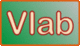online_pump_pipe_system:  Operating discharge and head in a pump-pipe systemDefinition sketch of a pump-pipe system

 hp = a + bQ2
 H = c - dQ2

 INPUT DATA: [How to use] [Table of loss coefficients]

Select:

 SI units (metric) U.S. Customary units
Pipe diameter D :

Pipe length L :

Friction factor f :

Elevation E1 :

Elevation E2 :

Entrance loss coefficient Ke :        [Leave blank to set default Ke = 0.5]
Exit loss coefficient KE :        [Leave blank to set default KE = 1]
Sum of bend loss coefficient(s) [zero or more bends] Kb :

Select type of pump head-discharge relation H vs Q:
 1. Equation 2. Array

If type (of H vs Q) = 1, enter c and d  in   H = c - dQ2:

c :

d :

Else, if type (of H vs Q) = 2, enter N and H and Q arrays below:

Number of N  values in pump head-discharge relation H vs Q :

N  paired values of H and Q (separate each value with a comma; string length
75 characters, maximum 150).

H :

Q :

OUTPUT:

The system operating point is at the intersection of system and pump head-discharge relations.

 Operating discharge: Q =  0   Operating head: h =  0

 [Click on figure to expand]Pump on Wikipedia

Sample
Input-Output

Your request was processed at  09:50:38 pm on June 30th, 2022   [ 220630  21:50:38 ].

Thank you for running online_pump_pipe_system.